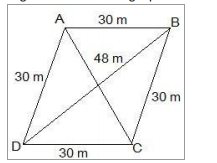# NCERT Solutions Class 9 Mathematics Solutions for Heron’s Formula - Exercise 12.2 in Chapter 12 - Heron’s Formula

A rhombus shaped field has green grass for 18 cows to graze. If each side of the rhombus is

30 m and its longer diagonal is 48 m, how much area of grass field will each cow be getting?

Draw a rhombus-shaped field first with the vertices as ABCD. The diagonal AC divides the

rhombus into two congruent triangles which are having equal areas. The diagram is as follows.Consider the triangle BCD,

Its semi-perimeter = (48 + 30 + 30)/2 m = 54 m

Using Heron's formula,

Area of the ΔBCD =

\begin{aligned} &\sqrt{s(s-a)(s-b)(s-c)}\\ &(\sqrt{54(54-48)(54-30)(54-30)}) m^{2}\\ &\left.=432 \mathrm{m}^{2} \times 6 \times 24 \times 24\right) \mathrm{m}^{2}\\ &\text { Area of field }=2 \times \text { area of the } \Delta B C D=(2 \times 432) \mathrm{m}^{2}=864 \mathrm{m}^{2} \end{aligned}

Thus, the area of the grass field that each cow will be getting = (864 / 18) \mathrm{m}^{2}=48 \mathrm{m}^{2}

Video transcript
"hello students welcome to leader q a video session i am saf your max tutor and question for today is a rhombus shaped field has green grass for 18 cows to graze if each side of the rhombus is 30 meter and its longer diagonal is 48 meter how much area of grass field will each cow be getting so first of all you draw the rhombus shape field with the vertices as shown in the figure so this rhombus abcd is a field as per the question and you can see inside this rhombus diagonal ac is there which divides the rhombus into two congruent triangles and these triangles are triangle adb and triangle bdc or bcd now consider triangle bcd inside this triangle first of all let us find semi parameter to find its area so s will be equal to 48 sum all the side and then divided by 2 so s will be equal to 54 meter now use the harrows formula to find the area so as per the harrows formula you know let it indicate as a that is area of the triangle so area will be equal to under root of s s minus a s minus b as minus c let us substitute value of a b c and s so this will give you as is 54 54 minus 48 and then 54 minus 30 and then 54 minus 30 again so this is our arrows formula applied we will continue over here so area comes out to be a is equal to 432 meter square and hence area of the field will be 2 into area of triangle bcd because this field is made up of two triangles which are congruent as these two triangles are congruent their area will also be same so you can say that this area of triangle abd will be equal to area of triangle bcd so you can see here that the area of the field will be equal to 2 into area of triangle bc d that is 2 into 432 the area will be equal to 864 meter square thus the area of the grass filled at each cow that each cow will be great grazing will be equal to 864 meter square divided by 18 as you can see there are 18 cows we have to divide it by 18 for finding the area grazed by each cow 864 divided by 18 and that comes out to be 48 square and that is our final answer for the area area of the grass grazed by each cow if you have any query you can post it in our comment section and subscribe to lido for more such q and a thank you for watching "
Related Questions

Lido

Courses

Teachers

Book a Demo with us

Syllabus

Maths
CBSE
Maths
ICSE
Science
CBSE

Science
ICSE
English
CBSE
English
ICSE
Coding

Terms & Policies

Selina Question Bank

Maths
Physics
Biology

Allied Question Bank

Chemistry
Connect with us on social media!